# SUMIFS Excel FunctionArticle byJeevan A Y## What Is SUMIFS Function In Excel?

The SUMIFS Excel function allows users to sum values in matching cells that meet multiple conditions, such as number, text, date, logical operators, wildcards, etc. Unlike SUMIF, the SUMIFS function allows users to specify up to 127 criteria (ranges).

For instance, we have the regional sales data of salespersons.

Assuming we need to get the total sales made by John in East. First, we will create criteria (reference) cells for both John and East in E and F columns. The criteria given here are Salesperson, i.e., John and Region, i.e., East. Next, we will enter the following formula into cell G2.

=SUMIFS(C2:C10,A2:A10,E2,B2:B10,F2)

We have the total sales for the salesperson “John” and the region “East” as \$9,019 in cell G2.

###### Key Takeaways
• SUMIFS function is used to sum values in cells based on the multiple criteria.
• It can also be used with logical operators, like equal (=), not equal (< >), greater than or equal to (>=), and less than or equal to (<=).
• The function supports wildcards, like an asterisk (*) and question mark (?) for partial matching.
• SUMIFS gives only numerical values as output. Therefore, all the blank cells and text values will be ignored in the calculation. However, Boolean value TRUE will be considered 1 and FALSE as 0.

### SUMIFS() Excel Formula

The SUMIFS excel formula is nearly identical to the SUMIF function, but requires additional parameters to match multiple criteria.

=SUMIFS(sum_range, criteria_range1, criteria1, [criteria_range2, criteria2], …)

• Sum_Range: This is the range of cells we need to sum based on the criteria given in the subsequent arguments. Blank cells and text values will be ignored from the calculation. This is a mandatory argument.

• Criteria_Range1: This is the first range to evaluate using associated criteria 1. This is a mandatory argument.

• Criteria1: The first condition determines which cells to sum in the criteria range 1. It can be a cell reference, a number, a logical expression, a text string, or some other Excel formula. This is a mandatory argument.

• [Criteria_Range2]: This is the second range 2 to evaluate using associated criteria 2. This is an optional argument.

• [Criteria2]: The second condition determines which cells to sum in the given criteria range 2. This is an optional argument.

### How To Use SUMIFS Function?

Let us look at a basic SUMIFS function in Excel example before moving to advanced ones.

We have city-wise monthly sales data for a food factory.

We need to sum sales based on a particular city and category from the above data. First, create two criteria (reference) cells, as shown in the following image.

Following are the steps to apply the SUMIFS excel function to get the total sales:

1. Enter the SUMIFS function in cell I2.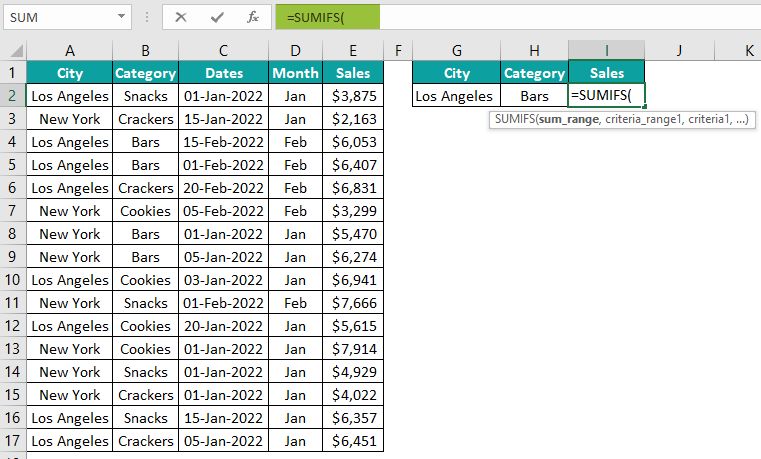2. Choose the sales column range E2:E17 for the first argument sum range.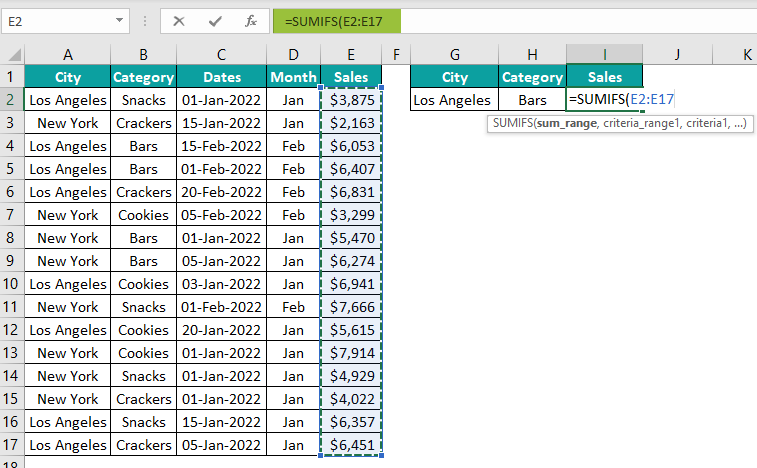3. The next argument is the criteria range 1, i.e., based on what criteria we are trying to sum sales. So, there are two criteria – City and Category.

Choose the city column range A2:A17 as Criteria Range 1.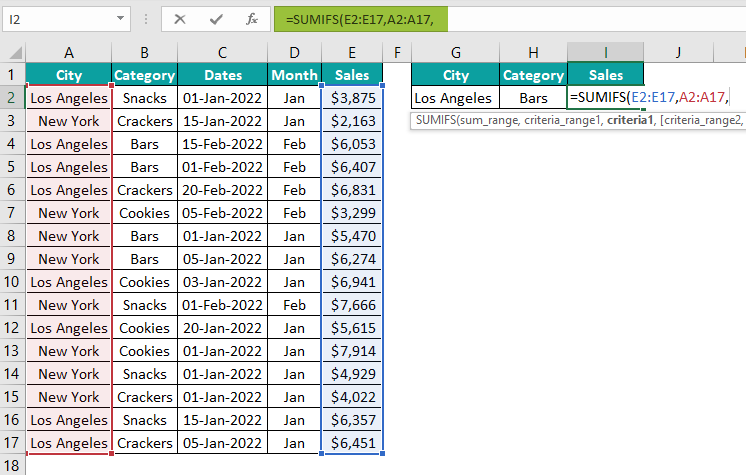4. The next argument is Criteria 1, i.e., the condition we will apply from the criteria range 1. Choose the city reference cell G2 for this.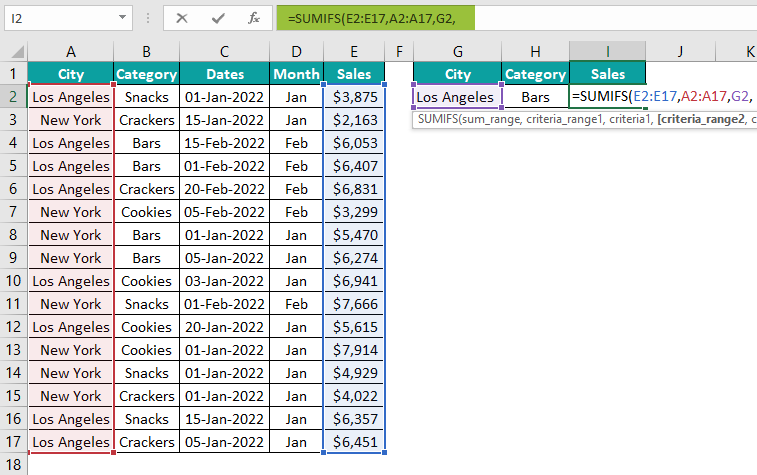5. Now, choose the Category column range B2:B17 for the criteria range 2.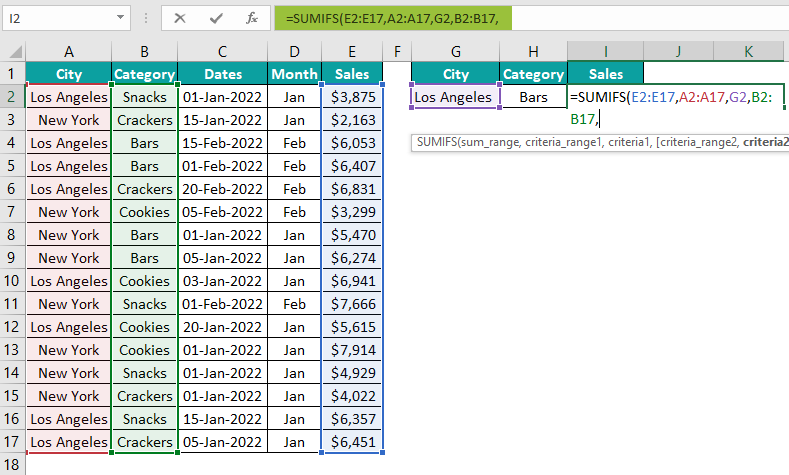6. For criteria 2, choose the category reference cell H2.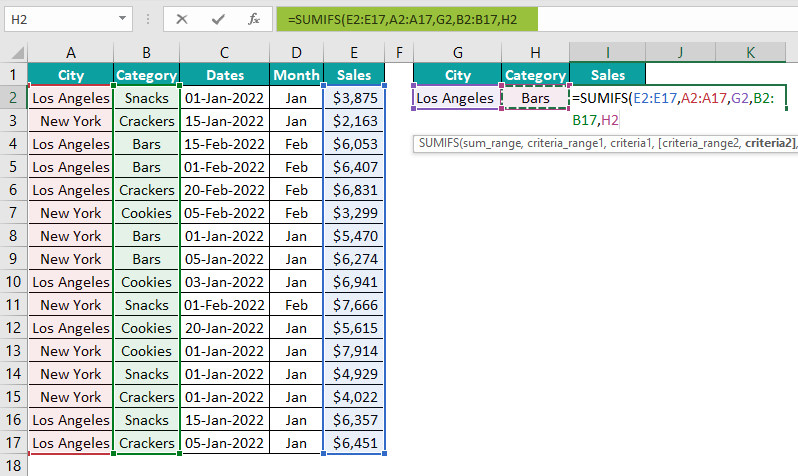7. We are looking into only 2 criteria. Close the bracket and hit the “Enter” key to get the result.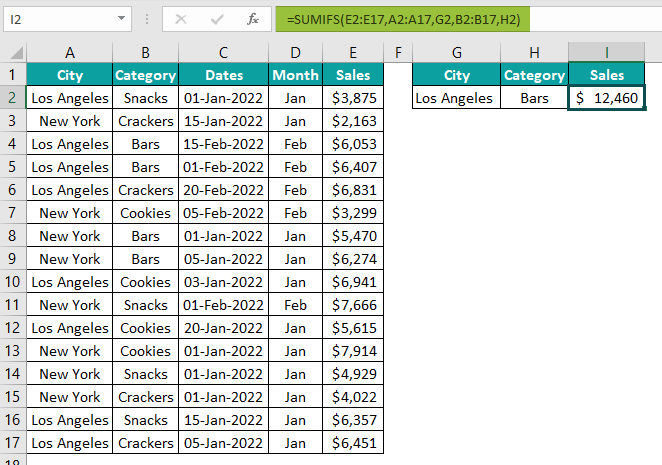The total sales for “Los Angeles” and the category “Bars” are \$12,460. SUMIFS function adds cells from E2:E17 by applying the city “Los Angeles” criteria in the range of cells A2:A17 and the category “Bars” in cells B2:B17.

### Examples

Let us look at some advanced SUMIFS function in excel to understand how it works:

#### Example 1: SUMIFS Function With Comparison Operators

The SUMIFS function not only allows users to sum cells for the specified criteria but also uses operators like greater than or equal to (>=) and less than or equal to (=<) operators for comparisons.

For example, we have item-by-item grocery store sales in an Excel spreadsheet below.

We will find the sum of units sold (greater than or equal to 55) for a given category using the above data. But, first, create two criteria (reference) cells as shown in the following image.

Step 1: Enter the SUMIFS function in cell H3.

Step 2: Enter the sum range as C2:C21.

Step 3: Enter the Category column range A2:A21 as criteria range 1.

Step 4: Set the criteria for criteria range 1, i.e., Category Name. So, enter reference cell H1.

Step 5: Next, choose criteria range 2, i.e., the Units Sold column from C2:C21.

Step 6: Now, set the criteria for criteria range 2, i.e., the units sold (>=55) in double quotes.

Close the bracket and hit the “Enter” key to get the result.

So, the total units sold (>=55) for the category “Cookies” is 214. Filter the data in the table and cross-check as shown in the following image.

There are 4 transactions, but SUMIFS considered only those when the units sold were >=55.

#### Example 2: SUMIFS Function With Dates

The SUMIFS function is also used with dates along with logical operators. For instance, we have the following sales data.

Assuming we need to calculate the total units sold for a particular salesperson within certain dates. Let us look at the use of SUMIFS function in Excel in this case.

We need to calculate the total units sold by Joe (criteria cell H1) in East (reference cell H2) and between dates 01-Jan-2022 to 31-Jan-2022 in cells H3 and H4, respectively.

So, we have multiple criteria to fulfill here.

Step 1: Enter the SUMIFS function in cell H5.

Step 2: Select the sum range from D2:D14.

Step 3: Next, we need to enter the criteria range one by one. The first will be the criteria range 1, i.e., the salesperson column A2:A14.

Step 4: The criteria for criteria range 1 will be the salesperson from the selected range, i.e., cell H1.

Step 5: Next criteria range 2 is the region, so select Regions column range C2:C14.

Step 6: Set the criteria for the criteria range 2, i.e., cell H2.

Step 7: Next, we need to apply the dates between the two ranges. So, select the Dates column range B2:B14 as the starting date criteria.

Step 8: From the selected dates, we need to start looking for sales on or after (greater than or equal to) 01-Jan-2022. So, enter the criteria as shown in the following image.

Step 9: Select dates from B2:B14 to give the ending date criteria.

Step 10: For this date, give the ending date as less than or equal to 31-Jan-2022.

All the criteria are applied. Then, close the bracket and hit the “Enter” key to get the result.

So, the total units sold by Joe in the East and between dates 01-Jan-2022 to 31-Jan-2022 is 1,431.

#### Example 3: Wildcard Criteria With The SUMIFS Function

The SUMIFS function also supports wildcards for partial matching. For instance, below is the employee salary data.

We have full names in the data table, but we need to get the total salary for all the employees whose names start with Smith in cell E2.

Step 1: Enter the SUMIFS function in cell E2.

Step 2: Enter the sum range from B2:B6.

Step 3: Enter the criteria range 1 from A2:A6.

Step 4: We need to combine the name Smith with the wildcard character asterisk (*) to set the criteria.

Here, the asterisk (*) matches any number of characters that come after Smith. Hence, this will match all the employees whose name starts with Smith.

Close the bracket and hit the “Enter” key to get the result.

The result in cell E2 is \$1,390.

Wildcard matched only for employees Smith Paula in cell A2 and Smith Steven in cell A5.

However, employee Robin Smith in cell A6 is not matched even though it has the name Smith. Smith is the ending name here, not the starting name.

To get the sum of employees whose name ends with Smith, we need to change the wildcard position from end to start.

Let us follow the same example.

As we can see, wildcard matches only Robin Smith in cell A6.

To match the start and end, we need to surround the asterisk (*) on either end of the criteria. As a result, it returned all the names that have Smith.

### Important Things To Note

• SUMIFS function, unlike SUMIF, is used to match more than one criteria.
• It can match up to 127 criteria.
• The function supports wildcards, like an asterisk (*) and question mark (?) for partial matching.
• Asterisk (*) will match any number of characters. For example, “*”&Smith will match all the characters before the name, while Smith&“*” will match any number of characters after the name.
• SUMIFS returns only numerical values.
• All the blank cells and text values will be ignored.
• The criteria length should not be longer than 255 characters.
• All the argument ranges should be the same size as the sum range.

What is SUMIFS function in Excel?

SUMIFS is an arithmetic function in Excel that can be used to sum cells based on multiple criteria.

How to use SUMIFS function in Excel with multiple criteria?

SUMIFS supports up to 127 criteria.

For instance, the following is the sales data.If we want to get the sum of quantity for the region “East”, for the city “Boston”, for the category “Crackers”, and the product “Whole Wheat”, we can use the SUMIFS function.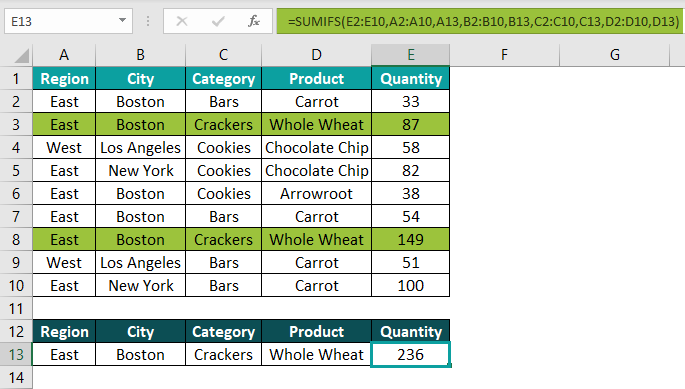In the above example, SUMIFS evaluated 4 different criteria and only returned the sum for the matching criteria rows.

When to use SUMIFS function in Excel?

Whenever you want to sum something based on more than one criteria, you can use the SUMIFS function.

Where to find SUMIFS function in Excel?

SUMIFS function in Excel is a Math & Trigonometry function located under the Formula bar.You can also type the equal or plus sign in any of the cells and start typing SUMIFS to enter the function.